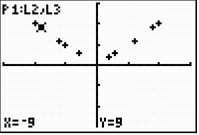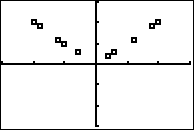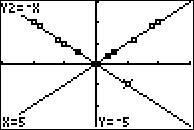••• ##### Device
• TI-83 Plus Family
• TI-84 Plus
• TI-84 Plus Silver Edition
•TI-84 Plus C Silver Edition
•TI-84 Plus CE
• ##### Report an Issue

Algebra I: Introducing the Absolute Value Function
by Texas Instruments#### Overview

Students will examine data by comparing individual data points to the mean by finding the difference (positive or negative) and the distance from the mean.

#### Key Steps

•Students will examine data by comparing individual data points to the mean by finding the difference (positive or negative) and the distance from the mean. Then Students will plot the distances versus the differences to examine the shape of the plot.

•Students will investigate the absolute value function in the Y= register to model the relationship between the distances and the differences. Then, students will extend the investigation of absolute value equations by examining tables and graphs.